Courses

# Test: Permutation, Combination & Probability - 2 (2017-2010)

## 15 Questions MCQ Test UPSC Topic Wise Previous Year Questions | Test: Permutation, Combination & Probability - 2 (2017-2010)

Description
This mock test of Test: Permutation, Combination & Probability - 2 (2017-2010) for UPSC helps you for every UPSC entrance exam. This contains 15 Multiple Choice Questions for UPSC Test: Permutation, Combination & Probability - 2 (2017-2010) (mcq) to study with solutions a complete question bank. The solved questions answers in this Test: Permutation, Combination & Probability - 2 (2017-2010) quiz give you a good mix of easy questions and tough questions. UPSC students definitely take this Test: Permutation, Combination & Probability - 2 (2017-2010) exercise for a better result in the exam. You can find other Test: Permutation, Combination & Probability - 2 (2017-2010) extra questions, long questions & short questions for UPSC on EduRev as well by searching above.
QUESTION: 1

### A bag contains 20 balls. 8 balls are green, 7 are white and 5 are red. What is the minimum number of balls that must be picked up from the bag blindfolded (without replacing any of it) to be assured of picking at least one ball of each colour? 

Solution:

Considering the worst case First 15 picked balls are red and white. Hence in 16th time it is assured that 3 different colour balls exist.

QUESTION: 2

Solution:
QUESTION: 3

### A person ordered 5 pairs of black socks and some pairs of brown socks. The price of a black pair was thrice that of a brown pair. While preparing the bill, the bill clerk interchanged the number of black and brown pairs by mistake which increased the bill by 100%. What was the number of pairs of brown socks in the original order? 

Solution:

Let number of a pairs of  brown socks = y
Price of brown socks = x
Price of black socks = 3x
According to question
⇒ 5 x 3x + yx = 100   ... (i)
Now, clerk has interchanged socks pairs then price is increased by 100%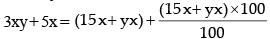⇒ 3xy + 5x = 30x + 2xy ... (ii)
⇒ 30x + 2yx = 3xy + 5x
⇒ 25x = xy
y = 25
∴ So, number of  brown socks = 25

QUESTION: 4

A student has to opt for 2 subjects out of 5 subjects for a course, namely, Commerce, Economics, Statistics, Mathematics I and Mathematics II. Mathematics II can be offered only if Mathematics I is also opted. The number of different combinations of two subjects which can be opted is



Solution:

It mathematics I is not opted, then two subjects out of four subjects have to be opted for.
∴ Number of ways in which two subjects can be opted for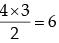If mathematics II is opted, then it can be offered only if mathematics I is also opted for Number of ways in which two subjects can be opted for = 6 +1 = 7.

QUESTION: 5

A selection is to be made for one post of Principal and two posts of Vice-Principal. Amongst the six candidates called for the interview, only two are eligible for the post of Principal while they all are eligible for the post of Vice-Principal. The number of possible combinations of selectees is



Solution:

Number of ways to select Principal = 2C1
Number of ways to select Vice Principal = 5C2
Total number of ways = 2C1 + 5C2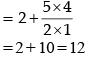Number of possible combinations of selectres = 2 × 10 = 20

QUESTION: 6

In a box of marbles, there are three less white marbles than the red ones and five more white marbles than the green ones. If there are a total of 10 white marbles, how many marbles are there in the box?



Solution: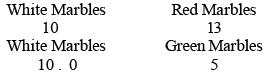Now, total number of Marbles = 5 + 10 + 13 = 28

QUESTION: 7

In a society it is customary for friends of the same sex to hug and for friends of opposite sex to shake hands when they meet. A group of friends met in a party and there were 24 handshakes. Which one among the following numbers indicates the possible number of hugs?



Solution:

Let x be the number of women.
Let y be the number of men.
Total number of hand shakes = xy = 24
Then, the possible factors of x and y are x = 6 or  4, y = 4 or 6
Number of hugs = xC2+ yC2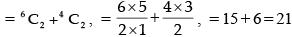QUESTION: 8

There are 5 tasks and 5 persons. Task-l cannot be assigned to either person-l or person-2. Task-2 must be assigned to either person-3 or person-4. Every person is to be assigned one task. In how many ways can the assignment be done?



Solution:

Here are five persons, and 5 tasks
So, When T2 task is fixed for person 3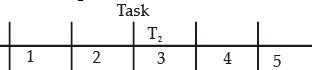For Task 1 no. of ways = 2
Task 2 no. of ways = 1
Task 3 no. of ways = 3
Task 4 no. of ways = 3
Task 5 no. of ways = 3
Total no. of ways for condition = 3 + 3 + 3 + 2 + 1 = 12
Condition II
When task T2 is given to be person 4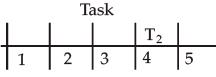No. of ways for Task T1 = 2
No. of ways for Task T2 = 1
No. of ways for Task T3 = 3
No. of ways for Task T4 = 3
No. of ways for Task T5 = 3
Total number of ways for condition II
= 3 + 3 + 3 + 2 +1 = 12
Total number of ways for condition I and II = 12 + 12 = 24

QUESTION: 9

Twelve people form a club. By picking lots, one of them will host a dinner for all once in a month. The number of dinners a particular member has to host in one year is



Solution:

We cannot predict the number of dinners for a particular member from the given data. It may be possible that by choosing members from picking lots, one may have to host a dinner more than one times.

QUESTION: 10

A group of 630 children is seated in rows for a group photo session. Each row contains three less children than the row in front of it. Which one of the following number of rows is not possible?



Solution:

Let no. of column = x, no. of rows = y
∴ xy = 630 – [3 × 1 + 3 × 2 + ......... + 3 × (y – 1)]
= 630 – 3 [1 + 2 + ........ + (y – 1)]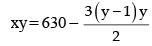(a) If y = 3, then 3x = 630 – 9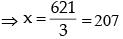(b) If y = 4, then 4x = 630 – 18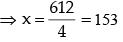(c) If y = 5, then 5x = 630 – 30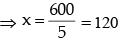QUESTION: 11

There are 100 students in a particular class. 60% students play cricket, 30% student play football and 10% students play both the games. What is the number of students who play neither cricket nor football?



Solution:

Given, n (U) = 100
Number of students who play cricket = 60
i.e. n(C) = 60
Number of students who play football = 30
i.e. n(F) = 30
Number of students who play both the games = 10
i.e. n(C ∩ F) = 10
To find : n(C' ∩ F') = ?
we know,
n(C ∪ F) = n(C) + n(F) – n(C ∩ F)
= 60 + 30 –10 = 80
n(C' ∩ F') = n(C ∪ F)' = n(U) – n(C ∩ F)
= 100 – 80 = 20

QUESTION: 12

In how many ways can four children be made to stand in a line such that two of them, A and B are always together?



Solution:

Take, A and B to be always together as a single entity.
Now, total no. of children = 4 – 2 + 1 = 3
These can be arranged in 3! ways and A, B can be arranged among themselves in 2! ways.
Hence, no. of arrangements such that A and B are always together = 3! × 2! = 3 × 2 × 2 = 12

QUESTION: 13

When ten persons shake hands with one another, in how many ways is it possible?



Solution:

First person can shake hand with the other 9 i.e, in 9 ways. Second person can shake hand with the remaining 8 persons and so on.
∴ total no. of hands shaked = 9 + 8 + ....... + 2 + 1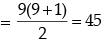QUESTION: 14

A person X has four notes of Rupee 1, 2, 5 and 10 denomination. The number of different sums of money she can form from them is



Solution:

Different sums of money can be formed by taking one, two, three and all the four notes together.
No. of different sums = 4C1 + 4C2 + 4C3 + 4C4
= 4 + 6 + 4 + 1 = 15

QUESTION: 15

A question paper had ten questions. Each question could only be answered as True (T) or False (F). Each candidate answered all the questions. Yet, no two candidates wrote the answers in an identical sequence. How many different sequences of answers are possible?



Solution:

Each question can be answered in 2 ways.
Hence, total no. of sequences = 2 × 2 × ........ 10 times.
= 210 = 1024